# Data Types in C#

## Data Types in C# with Examples

In this article, I am going to discuss the Data Types in C# with Examples. Please read our previous article where we discussed the Console Class Methods and Properties in C#. As a developer, it is very important to understand the Data Type in C#. This is because you need to decide which data type to use for a specific type of value.

##### Data Types:

Now let us understand what are the different data types available in .NET and in which scenario which data type is suitable in C#. Why I am going to keep the focus on this is that most of the time .NET Developers use limited data types. See, you will see that most of the time as a .NET developer, we are acquainted to use int, bool, double, string, and Datetime data types. These five data types are mostly used by .NET Developers. Because of the limited use of data types, we lose in terms of optimization and performance. So, at the end of this article, you will understand what are the different data types available in .NET and in which scenario you need to use which data types.

##### Why do we need Data Types in C#?

The Datatypes in C# are basically used to store the data temporarily in the computer through a program. In the real world, we have different types of data like integers, floating-point, characters, boolean, strings, etc. To store all these different kinds of data in a program to perform business-related operations, we need the data types.

##### What is a Data Type in C#?

The Datatypes are something that gives information about

1. Size of the memory location.
2. The Range of data that can be stored inside that memory location
3. Possible Legal Operations that can be performed on that memory location.
4. What Types of Results come out from an expression when these types are used inside that expression?

The keyword which gives all the above information is called the data type in C#.

##### What are the Different Types of Data types Available in C#?

A data type in C# specifies the type of data that a variable can store such as integer, floating, boolean, character, string, etc. The following diagram shows the different types of data types available in C#.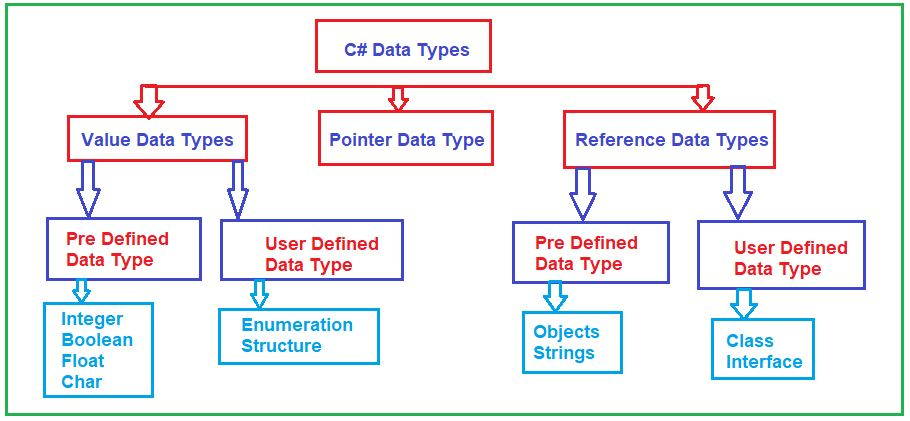There are 3 types of data types available in the C# language.

1. Value Data Types
2. Reference Data Types
3. Pointer Data Types

Let us discuss each of these data types in detail

##### What is Value Data Type in C#?

The data type which stores the value directly in the memory is called the Value Data Type in C#. The examples are int, char, boolean, and float which store numbers, alphabets, true/false, and floating-point numbers respectively. If you check the definition of these data types then you will see that the type of all these data types is going to be a struct. And struct is a value type in C#. The value data types in C# again classified into two types are as follows.

1. Predefined Data Types – Example includes Integer, Boolean, Boolean, Long, Double, Float, etc.
2. User-defined Data Types – Example includes Structure, Enumerations, etc.

Before understanding how to use data types in our programming language, let us first understand, how data is represented in a computer.

##### How Data is Represented in a Computer?

Before going and discussing how to use data types, first, we need to understand How data is represented on a computer? Let us understand this. Please have a look at the below diagram. See, on your computer’s hard disk, you have some data, let’s say A. The data can be in different formats, it can be an image, it can be an alphabet, it can be digits, it can be a PDF File, etc. Let us assume that you have some data called A. Now, we know the computer can only understand binary numbers i.e. 0’s and 1’s. So, the letter A is represented in the computer as 8 bits i.e. 01000001 (65 is the ASCII Value is A and hence the decimal number 65 is converted to its binary equivalent binary representation which is 01000001). So, the 0’s and 1’s are called bits. So, to store any data on the computer we need this 8-bit format. And this complete 8-bit is called a Byte. Now, as a dot net developer, it is very difficult for us to represent the data in binary format i.e. using 0s and 1s. So, here, in C# language we can use the decimal format. So, what we can do is, we will convert binary to decimal format, and internally the computer will map the decimal format to byte format (binary format), and then by using the byte we can represent the data. So, you can observe the byte representation of decimal number 65 is 01000001.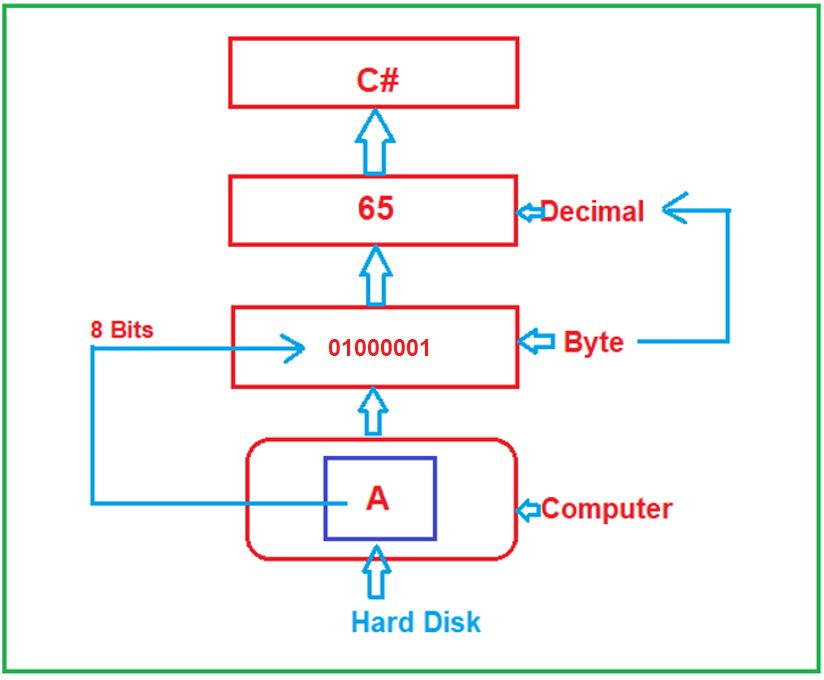Now, let us proceed and try to understand the different data types provided by .NET Framework to be used with C# Programming Language.

##### What is Byte Data Type in C#?

It is a .NET Data Type that is used to represent an 8-Bit unsigned integer. So, here, you might have one question i.e. what do you mean by unsigned? Unsigned means only positive values. As it represents an 8-bit unsigned integer, so it can store 28 i.e. 256 numbers. As it stores only positive numbers, so the minimum value it can store is 0 and the maximum value it can store is 255. Now, if you go to the definition of byte, then you will see the following.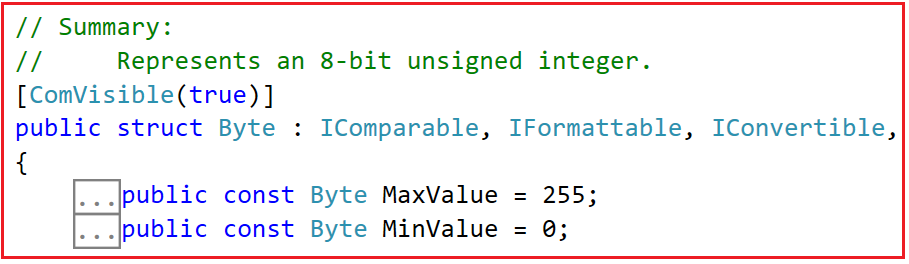Now, if you want to store both positive and negative values, then you need to use the signed byte data type i.e. SByte. This SByte data type represents an 8-bit signed integer. Then what will be the maximum and minimum values? Remember when a data type is signed, then it can hold both positive and negative values. In that case, the maximum needs to be divided by two i.e. 256/2 which is 128. So, it will store 128 positive numbers and 128 negative numbers. So, in this case, the positive numbers will be from 0 to 127 and the negative numbers will be from -1 to -128. For a better understanding, please go to the definition of SByte data type and you will see the following.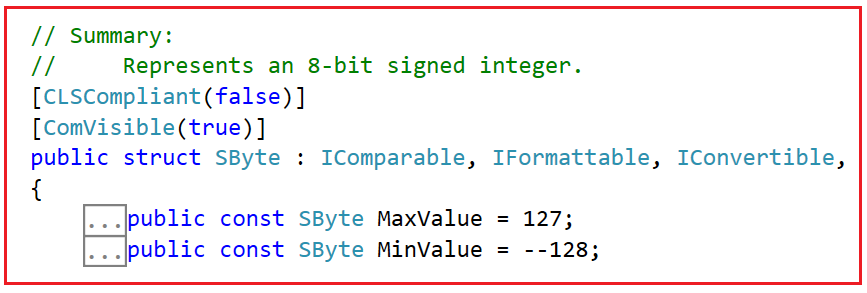##### ASCII Code:

To understand the byte data type in detail, we need to understand something called ASCII code. Please visit the following link to understand the ASCII Codes. ASCII stands for American Standard Code for Information Interchange.

https://www.cs.cmu.edu/~pattis/15-1XX/common/handouts/ascii.html

When you visit the above site, you will get the following table which shows the Decimal Number and its equivalent character or symbol.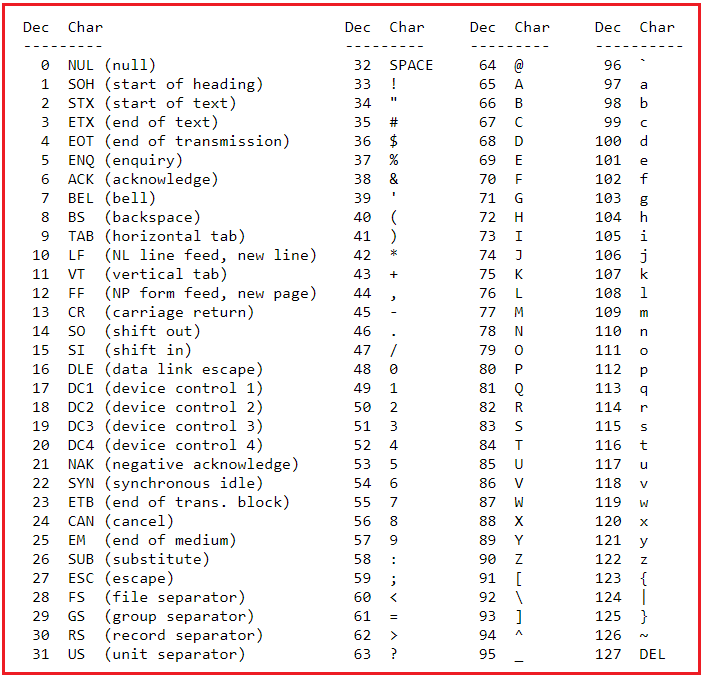We have already discussed how to convert Decimal to Binary numbers. Now, suppose you want to store decimal number 66, whose binary representation i.e. 1000010. And you can see in the above table that the capital letter B is the character equivalent of 66.

##### Example to understand Byte and SByte Data Types in C#:

Please have a look at the below example to understand the Byte and SByte data type in C#. The following example code is self-explained, so please go through the comment lines. In the below example, we are showing what value or data we can store and we cannot store and we are also showing what is the maximum and minimum value that we can store in the Byte and SByte variable as well as we are showing the size of the Byte and SByte data types. We are also printing the size of the byte data type using the sizeof operator.

```using System;
namespace DataTypesDemo
{
class Program
{
static void Main(string[] args)
{
byte b1 = 66;
//You cannot store negative number using byte data type.
//The following statement will give compile time error
//byte b2 = -100;

//The following Statement will give compile time error
//The maximum value you can store in a byte variable is 255
//byte b3 = 256;

Console.WriteLine(\$"Decimal: {b1}");
Console.WriteLine(\$"ASCII Equivalent Character of {b1} is {(char)b1}");
Console.WriteLine(\$"Byte Min Value:{sbyte.MinValue} and Max Value:{sbyte.MaxValue}");
Console.WriteLine(\$"Byte Size:{sizeof(sbyte)} Byte");

sbyte sb1 = 66;
//You can store negative number using sbyte data type.
//The following statement will not give compile time error
sbyte sb2 = -100;

//The following Statement will give compile time error
//The maximum value you can store in a sbyte variable is 128
//sbyte sb3 = 128;

//The following Statement will give compile time error
//The minimum value you can store in a sbyte variable is -128
//sbyte sb4 = -129;

Console.WriteLine(\$"\nDecimal: {sb1}");
Console.WriteLine(\$"ASCII Equivalent Character of {sb1} is {(char)sb1}");
Console.WriteLine(\$"ASCII Equivalent Character of {sb2} is {(char)sb2}");
Console.WriteLine(\$"SByte Min Value:{sbyte.MinValue} and Max Value:{sbyte.MaxValue}");
Console.WriteLine(\$"SByte Size:{sizeof(sbyte)} Byte");

}
}
}
```
###### Output: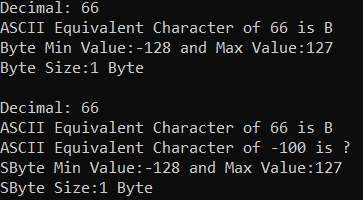Note: The most important point that you need to remember, if you want to represent 1-byte unsigned integer number, then you need to use the Byte data type in C#. In other words, we can also say that, if you want to store numbers from 0 to a maximum of 255 or the ASCII value of these numbers, then you need to go for Byte data type .NET Framework. And if you want to store numbers from -128 to 127 or their equivalent ASCII values then you need to use the SByte data type.

##### What is a char data type in C#?

Char is a 2-Byte length data type that can contain Unicode data. What is Unicode? Unicode is a standard for character encoding and decoding for computers. We can use various Unicode encodings formats such as UTF-8(8-bit), UTF-16(16-bit), and so on. As per the definition of char, it represents a character as a UTF-16 code unit. UTF-16 means 16-bit length which is nothing but 2-Bytes.

Again, it is a signed data type which means it can store only positive numbers. If you go to the definition of char data type, you will see the Maximum and Minimum values as follows.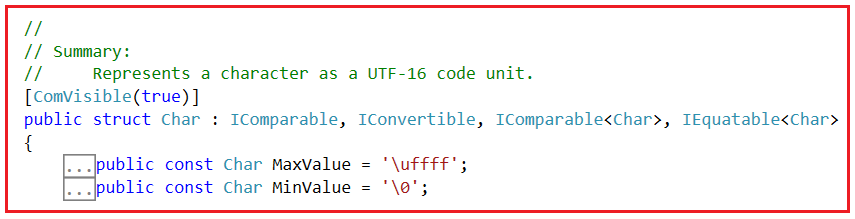Here, the ASCII symbol ‘\uffff’ represents 65535 and ‘\0’ represents 0. As char is 2-Byte length, so it will contain 216 numbers i.e. 65536. So, the minimum number is 0 and the maximum number is 65535. For a better understanding, please have a look at the below example.

```using System;
namespace DataTypesDemo
{
class Program
{
static void Main(string[] args)
{
char ch = 'B';
Console.WriteLine(\$"Char: {ch}");
Console.WriteLine(\$"Equivalent Number: {(byte)ch}");
Console.WriteLine(\$"Char Minimum: {(int)char.MinValue} and Maximum: {(int)char.MaxValue}");
Console.WriteLine(\$"Char Size: {sizeof(char)} Byte");

}
}
}
```
###### Output: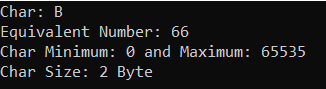Now, you might have one question. Here, we are representing the letter B using the char data type which is taking 2 Bytes. We can also represent this letter B using byte data type which is taking 1 Byte. Now, if byte and char are doing the same thing, then why do we need char data type which is taking some extra 1 Byte of memory?

##### Why Char Data Type in C#?

See, using byte data type we can only represent a maximum of 256 characters or you can say ASCII values. Byte will hold a maximum of 256 symbols/character, after 256 symbols/character, if we want to store some extra symbols like the Hindi alphabet, Chinese alphabet, or some special symbols which are not part of ASCII Characters, then it is not possible with the byte data type, because we already store the maximum symbols or characters. So, char is a Unicode character representation, it is having 2 Byte length and hence we can store the regional symbols, extra symbols, and special characters using the char data type in C#.

So, in other words, the byte is good if you are doing ASCII representation. But if you are developing a multilingual application, then you need to use Char Data Type. Multilingual application means applications that support multiple languages like Hindi, Chinese, English, Spanish, etc.

Now, you may have a counterargument that why not always use the char data type instead of byte data type because char is 2 bytes and it can store all the symbols available in the world. Then why should I use the byte data type? Now, remember char is basically used to represent Unicode characters. And when we read char data, internally it does some kind of transformations. And there are some scenarios where you don’t want to do such a kind of transformation or encoding.

Let’s say you have a raw image file. The raw image file has nothing to do with those transformations. In scenarios like this, we can use the Byte data type. There is something called a byte array you can use in situations like this.

So, the byte data type is good if you are reading the raw data or binary data, or the data without doing any kind of transformations or encoding. And char data type is good when you want to represent or show multilingual data or Unicode data to the end user.

To see, the list of UNICODE characters, please visit the following site.

https://en.wikipedia.org/wiki/List_of_Unicode_characters

##### String Data Type in C#:

In the previous example, we discussed the char data type where we are storing a single character in it. Now, if I try to add multiple characters to a char data type, then I will get a compile time error as shown in the below image.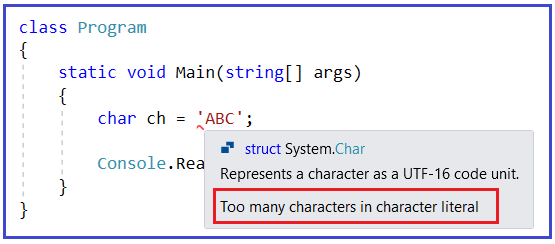As you can see, here we are getting the error Too many characters in character literal. This means you cannot store multiple characters in the character literal. If you want to store multiple characters, then you need to use the string data type in C# as shown in the below example,

```using System;
namespace DataTypesDemo
{
class Program
{
static void Main(string[] args)
{
string str = "ABC";
}
}
}
```

A string is nothing but a series of char data types. Now, you might have one question, how to know the size of a string? It’s very simple, first, you need to know the length of the string i.e. how many characters are there and then you need to multiply the length with the size of the char data type as a String is nothing but a series of char data types. For a better understanding, please have a look at the below example.

```using System;
namespace DataTypesDemo
{
class Program
{
static void Main(string[] args)
{
string str = "ABC";
var howManyBytes = str.Length * sizeof(Char);

Console.WriteLine(\$"str Value: {str}");
Console.WriteLine(\$"str Size: {howManyBytes}");

}
}
}
```
###### Output: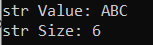In C#, the string is a reference type data type. Now, if you go to the definition of string data type, then you will see that the type is going to be a class as shown in the below image and the class is nothing but a reference type in C#.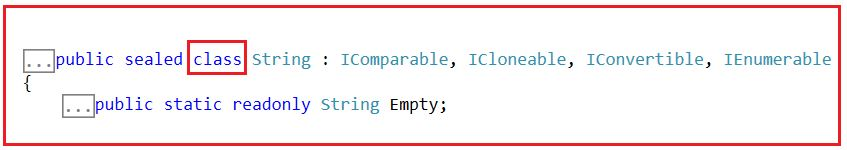Note: In our upcoming article, we will discuss more on strings like why strings are Reference Types, understanding the difference between the string (small) vs String (Capital), why strings are immutable, what is String intern, StringBuilder vs String for concatenation, string functions, and properties, etc.

##### Numeric Data Types in C#:

As of now, we have discussed Byte, SByte, Char, and String data types, which are going to store textual data. In other words, they can store numeric and non-numeric data. Using Byte and SByte we can store ASCII characters (Decimal Value of ASCII Characters). Using Char data type, we can store Unicode Characters (Decimal Value of Unicode Characters), and using string we can store textual data.

Now, let us proceed and try to understand how to store numeric data only. See, we have two types of numeric data. One with the number with a decimal point and another with a number without the decimal point.

##### Numbers without Decimal:

In this category, .NET Framework provided three kinds of data types. They are as follows:

1. 16-Bit Signed Numeric: Example: Int16
2. 32-Bit Signed Numeric: Example: Int32
3. 64-Bit Signed Numeric: Example: Int64

As the above data types are signed data types, so they can store both positive and negative numbers. Based on the data type, the size they can hold is going to vary.

##### 16-Bit Signed Numeric (Int16)

As it is 16-Bit, so it is going to store 216 numbers i.e. 65536. As it is signed, so it is going to store both positive and negative values. So, we need to divide 65536/2 i.e. 32,768. So, it is going to store 32,768 positive numbers as well as 32,768 negative numbers. So, the positive numbers will start from 0 up to 32,767 and the negative numbers will start from -1 up to -32,768. So, the minimum value this data type can hold is -32,768 and the maximum value this data type can hold is 32,767. If you go to the definition of Int16, you will see the following.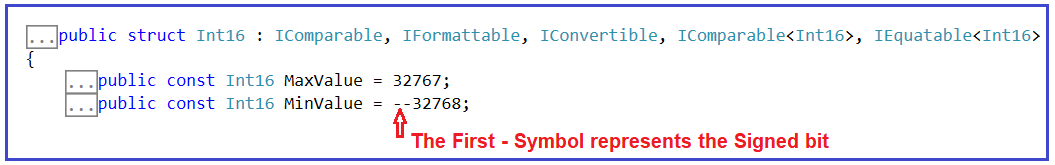##### 32-Bit Signed Numeric (Int32)

As it is 32-Bit, so it is going to store 232 numbers i.e. 4,294,967,296. As it is signed, so it is going to store both positive and negative values. So, we need to divide 4,294,967,296/2 i.e. 2,14,74,83,648. So, it is going to store 2,14,74,83,648 positive numbers as well as 2,14,74,83,648 negative numbers. So, the positive numbers will start from 0 up to 2,14,74,83,647 and the negative numbers will start from -1 up to -2,14,74,83,648. So, the minimum value this data type can hold is -2,14,74,83,648 and the maximum value this data type can hold is 2,14,74,83,647. If you go to the definition of Int32, you will see the following.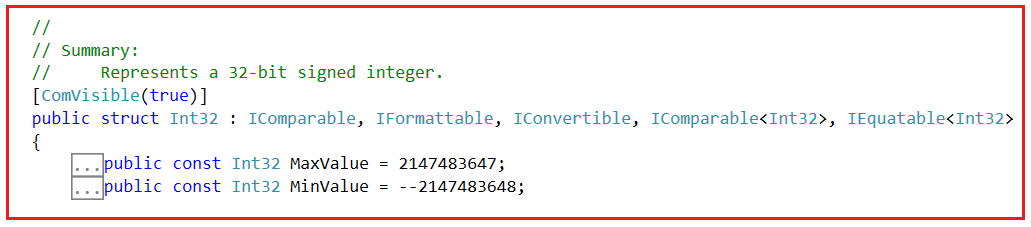##### 64-Bit Signed Numeric (Int64)

As it is 64-Bit, so it is going to store 264 numbers. As it is signed, so it is going to store both positive and negative values. I am not showing the ranges here as the values are going to be very big. If you go to the definition of Int64, you will see the following.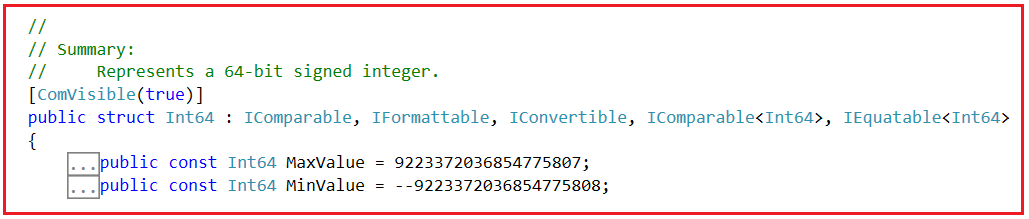Note: If you want to know the Max value and Min value of the Numeric data type, then you need to use MaxValue and MinValue field constants. If you want to know the size of the data type in bytes, then you can use sizeof function and to this function, we need to pass the data type (value type data type, not the reference type data type).

##### Example to Understand the Numeric Data Types without Decimal:
```using System;
namespace DataTypesDemo
{
class Program
{
static void Main(string[] args)
{
Int16 num1 = 123;
Int32 num2 = 456;
Int64 num3 = 789;

Console.WriteLine(\$"Int16 Min Value:{Int16.MinValue} and Max Value:{Int16.MaxValue}");
Console.WriteLine(\$"Int16 Size:{sizeof(Int16)} Byte");

Console.WriteLine(\$"Int32 Min Value:{Int32.MinValue} and Max Value:{Int32.MaxValue}");
Console.WriteLine(\$"Int32 Size:{sizeof(Int32)} Byte");

Console.WriteLine(\$"Int64 Min Value:{Int64.MinValue} and Max Value:{Int64.MaxValue}");
Console.WriteLine(\$"Int64 Size:{sizeof(Int64)} Byte");

}
}
}
```
###### Output: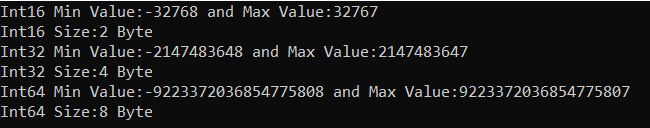One more important point that you need to remember is that these three data types can have other names as well. For example, Int16 can be used as a short data type. Int32 can be called an int data type and Int64 can be used as a long data type.

So, in our application, if we are using a short data type, it means it is Int16 i.e. 16-Bit Signed Numeric. So, we can use Int16 or short in our code and both are going to be the same. Similarly, if we are using int data type it means we are using Int32 i.e. 32-Bit Signed Numeric. So, we can use Int32 or int in our application code and both are going to be the same. And finally, if we are using long, it means we are using 64-Bit Signed Numeric. So, we can use Int64 or long in our code which is going to be the same. For a better understanding, please have a look at the below example.

```using System;
namespace DataTypesDemo
{
class Program
{
static void Main(string[] args)
{
//Int16 num1 = 123;
short num1 = 123;
//Int32 num2 = 456;
int num2 = 456;
// Int64 num3 = 789;
long num3 = 789;

Console.WriteLine(\$"short Min Value:{short.MinValue} and Max Value:{short.MaxValue}");
Console.WriteLine(\$"short Size:{sizeof(short)} Byte");

Console.WriteLine(\$"int Min Value:{int.MinValue} and Max Value:{int.MaxValue}");
Console.WriteLine(\$"int Size:{sizeof(int)} Byte");

Console.WriteLine(\$"long Min Value:{long.MinValue} and Max Value:{long.MaxValue}");
Console.WriteLine(\$"long Size:{sizeof(long)} Byte");

}
}
}
```
###### Output: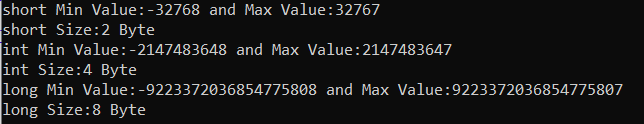Now, what if you want to store only positive numbers, the .NET Framework also provided the unsigned versions of each of these data types. For example, for Int16 there is UInt16, for Int32 there is UInt32, and for Int64, there is UInt64. Similarly, for short we have ushort, for int we have uint and for long we have ulong. These unsigned data types are going to store only positive values. The size of these unsigned data types is going to be the same as their signed data type. For a better understanding, please have a look at the following example.

```using System;
namespace DataTypesDemo
{
class Program
{
static void Main(string[] args)
{
//UInt16 num1 = 123;
ushort num1 = 123;

//UInt32 num2 = 456;
uint num2 = 456;

// UInt64 num3 = 789;
ulong num3 = 789;

Console.WriteLine(\$"ushort Min Value:{ushort.MinValue} and Max Value:{ushort.MaxValue}");
Console.WriteLine(\$"short Size:{sizeof(ushort)} Byte");

Console.WriteLine(\$"uint Min Value:{uint.MinValue} and Max Value:{uint.MaxValue}");
Console.WriteLine(\$"uint Size:{sizeof(uint)} Byte");

Console.WriteLine(\$"ulong Min Value:{ulong.MinValue} and Max Value:{ulong.MaxValue}");
Console.WriteLine(\$"ulong Size:{sizeof(ulong)} Byte");

}
}
}
```
###### Output: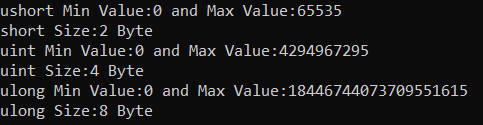As you can see in the above output, the min value of all these unsigned data types is 0 which means they are going to store only positive numbers without the decimal point. You can see, that when we use the unsigned data type, there is no division by 2 which is in the case of signed numeric data type.

##### When to use Signed and when to use unsigned data type in C#?

See, if you want to store only positive numbers, then it is recommended to use an unsigned data type, why because with a signed short data type the maximum positive number that you can store is 32767 but with unsigned ushort data type the maximum positive number you can store is 65535. So, using the same 2 Byes of memory, with ushort, we are getting a chance to store a bigger positive number as compared with the short data type positive number and the same will be in the case int and unit, long and ulong. If you want to store both positive and negative numbers then you need to use a signed data type.

##### Numeric Numbers with Decimal in C#:

Again, in Numbers with Decimal, we are provided with three flavors. They are as follows:

1. Single (single-precision floating-point number)
2. Double (double-precision floating-point number)
3. Decimal (Represents a decimal floating-point number)

The Single data type takes 4 Bytes, Double takes 8 Bytes and Decimal takes 16 Bytes of memory. For a better understanding, please have a look at the below example. In order to create a single value, we need to add the suffix f at the end of the number, similarly, if you want to create a Decimal value, you need to suffix the value with m (Capital or Small does not matter). If you are not suffixing with anything, then the value is going to be double by default.

```using System;
namespace DataTypesDemo
{
class Program
{
static void Main(string[] args)
{
Single a = 1.123f;
Double b = 1.456;
Decimal c = 1.789M;

Console.WriteLine(\$"Single Size:{sizeof(Single)} Byte");
Console.WriteLine(\$"Single Min Value:{Single.MinValue} and Max Value:{Single.MaxValue}");

Console.WriteLine(\$"Double Size:{sizeof(Double)} Byte");
Console.WriteLine(\$"Double Min Value:{Double.MinValue} and Max Value:{Double.MaxValue}");

Console.WriteLine(\$"Decimal Size:{sizeof(Decimal)} Byte");
Console.WriteLine(\$"Decimal Min Value:{Decimal.MinValue} and Max Value:{Decimal.MaxValue}");

}
}
}
```
###### Output: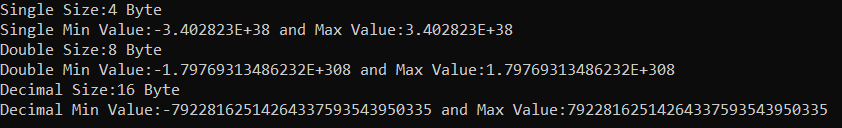Instead of Single, Double, and Decimal, you can also use the short-hand name of these data types such as float for Single, double for Double, and decimal for Decimal. The following example uses the short-hand names for the above Single, Double, and Decimal data types using C# Language.

```using System;
namespace DataTypesDemo
{
class Program
{
static void Main(string[] args)
{
float a = 1.123f;
double b = 1.456;
decimal c = 1.789m;

Console.WriteLine(\$"float Size:{sizeof(float)} Byte");
Console.WriteLine(\$"float Min Value:{float.MinValue} and Max Value:{float.MaxValue}");

Console.WriteLine(\$"double Size:{sizeof(double)} Byte");
Console.WriteLine(\$"double Min Value:{double.MinValue} and Max Value:{double.MaxValue}");

Console.WriteLine(\$"decimal Size:{sizeof(decimal)} Byte");
Console.WriteLine(\$"decimal Min Value:{decimal.MinValue} and Max Value:{decimal.MaxValue}");

}
}
}
```
###### Output: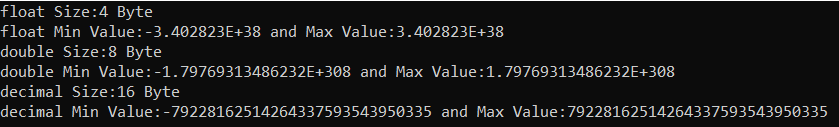##### Comparison between Float, Double, and Decimal:
###### Size:
1. Float uses 4 Bytes or 32 bits to represent data.
2. Double uses 8 Bytes or 64 bits to represent data.
3. Decimal uses 16 Bytes or 128 bits to represent data.
###### Range:
1. The float value ranges from approximately -3.402823E+38 to 3.402823E+38.
2. The double value ranges from approximately -1.79769313486232E+308 to 1.79769313486232E+308.
3. The Decimal value ranges from approximately -79228162514264337593543950335 to 79228162514264337593543950335.
###### Precision:
1. Float represents data with the single-precision floating-point number.
2. Double represents data with the double-precision floating-point numbers.
3. Decimal represents data with decimal floating-point numbers.
###### Accuracy:
1. Float is less accurate than Double and Decimal.
2. Double is more accurate than Float but less accurate than Decimal.
3. Decimal is more accurate than Float and Double.
###### Example to Understand Accuracy:

If you are using a float, then it will print a maximum of 7 digits, if you are using double, maximum it will print 15 digits and if you are using a decimal maximum, it will print 29 digits. For a better understanding, please have a look at the below example which shows the accuracy of float, double, and decimal data types in C# language.

```using System;
namespace DataTypesDemo
{
class Program
{
static void Main(string[] args)
{
float a = 1.78986380830029492956829698978655434342477f; //7 digits Maximum
double b = 1.78986380830029492956829698978655434342477; //15 digits Maximum
decimal c = 1.78986380830029492956829698978655434342477m; //29 digits Maximum

Console.WriteLine(a);
Console.WriteLine(b);
Console.WriteLine(c);

}
}
}```
###### Output: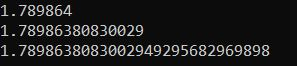##### Is it matter to choose the data type?

See, we can store a small integer number in a short data type, even we can store the same small integer number in a decimal data type. Now, you might be thinking that decimal or long data type accepts a bigger range of values, so always I will use these data types. Does it matter at all? Yes. It matters. What matters? Performance.

Let us see an example to understand how the data types impact the application performance in C# Language. Please have a look at the below example. Here, I am creating two loops that will be executed 100000 times. As part of the first for loop, I am using a short data type to create and initialize three variables with the number 100. In the second for loop, I am using decimal data type to create and initialize three variables with the number 100. Further, I am using StopWatch to measure the time taken by each loop.

```using System;
using System.Diagnostics;

namespace DataTypesDemo
{
class Program
{
static void Main(string[] args)
{
Stopwatch stopwatch1 = new Stopwatch();
stopwatch1.Start();
for(int i = 0; i <= 10000000; i++)
{
short s1 = 100;
short s2 = 100;
short s3 = 100;
}
stopwatch1.Stop();
Console.WriteLine(\$"short took : {stopwatch1.ElapsedMilliseconds} MS");

Stopwatch stopwatch2 = new Stopwatch();
stopwatch2.Start();
for (int i = 0; i <= 10000000; i++)
{
decimal s1 = 100;
decimal s2 = 100;
decimal s3 = 100;
}
stopwatch2.Stop();
Console.WriteLine(\$"decimal took : {stopwatch2.ElapsedMilliseconds} MS");

}
}
}
```
###### Output: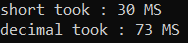So, you can see, short took 30 MS compared with 73 MS with decimal. So, it is matter, that you need to choose the right data type in your application development to get better performance.

##### How to Get the Size of PreDefined Data Types in C#?

If you want to know the actual size of pre-defined or built-in data types, then you can make use of sizeof method. Let’s understand this with an example. The following example gets the size of different predefined data types in C#.

```using System;
namespace DataTypesDemo
{
class Program
{
static void Main(string[] args)
{
Console.WriteLine(\$"Size of Byte: {sizeof(byte)}");
Console.WriteLine(\$"Size of Integer: {sizeof(int)}");
Console.WriteLine(\$"Size of Character: {sizeof(char)}");
Console.WriteLine(\$"Size of Float: {sizeof(float)}");
Console.WriteLine(\$"Size of Long: {sizeof(long)}");
Console.WriteLine(\$"Size of Double: {sizeof(double)}");
Console.WriteLine(\$"Size of Bool: {sizeof(bool)}");
}
}
}
```
###### Output: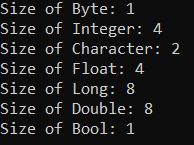##### How to Get the Minimum and Maximum Range of Values of Built-in Data Types in C#?

If you want to know the maximum and minimum range of numeric data types, then you can make use of the MinValue and MaxValue constants. If you go to the definition of each numeric data type, then you will see these two constants which hold the maximum and minimum range of values the data type can hold. For a better understanding, please have a look at the following example. In the below example, we are using the MinValue and MaxValue constants to get the data type’s maximum and minimum value range.

```using System;
namespace DataTypesDemo
{
class Program
{
static void Main(string[] args)
{
Console.WriteLine(\$"Byte => Minimum Range:{byte.MinValue} and Maximum Range:{byte.MaxValue}");
Console.WriteLine(\$"Integer => Minimum Range:{int.MinValue} and Maximum Range:{int.MaxValue}");
Console.WriteLine(\$"Float => Minimum Range:{float.MinValue} and Maximum Range:{float.MaxValue}");
Console.WriteLine(\$"Long => Minimum Range:{long.MinValue} and Maximum Range:{long.MaxValue}");
Console.WriteLine(\$"Double => Minimum Range:{double.MinValue} and Maximum Range:{double.MaxValue}");
}
}
}
```
###### Output: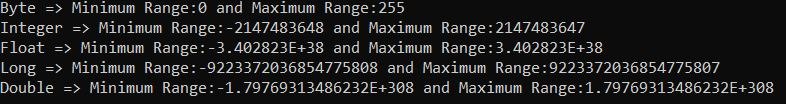##### How to Get the Default Values of built-in Data Types in C#?

Every built-in data type has a default value. All the numeric type has 0 as the default value, boolean has false, and char has ‘\0’ as the default value. You can use the default(typename) to know the default value of a data type in C#. For a better understanding, please have a look at the below example.

```using System;
namespace DataTypesDemo
{
class Program
{
static void Main(string[] args)
{
Console.WriteLine(\$"Default Value of Byte: {default(byte)} ");
Console.WriteLine(\$"Default Value of Integer: {default(int)}");
Console.WriteLine(\$"Default Value of Float: {default(float)}");
Console.WriteLine(\$"Default Value of Long: {default(long)}");
Console.WriteLine(\$"Default Value of Double: {default(double)}");
Console.WriteLine(\$"Default Value of Character: {default(char)}");
Console.WriteLine(\$"Default Value of Boolean: {default(bool)}");
}
}
}
```
###### Output: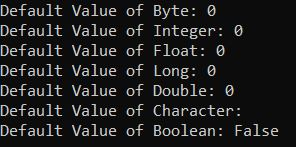##### What is the Reference Data Type in C#?

The data type which is used to store the reference of a variable is called Reference Data Type. In other words, we can say that the reference types do not store the actual data stored in a variable, rather they store the reference to the variables. We will discuss this concept in a later article. Again, the Reference Data Types are categorized into 2 types. They are as follows.

1. Predefined Types – Examples include Objects, Strings, and dynamics.
2. User-defined Types – Examples include Classes and Interfaces.
##### What is Pointer Type in C#?

The pointer in C# language is a variable, it is also known as a locator or indicator that points to an address of the value which means pointer-type variables stores the memory address of another type. To get the pointer details we have two symbols ampersand (&) and asterisk (*).

1. ampersand (&): It is known as Address Operator. It is used to determine the address of a variable.
2. asterisk (*): It is also known as Indirection Operator. It is used to access the value of an address.

For a better understanding, please have a look at the below example which shows the use of the Pointer data Type in C#. In order to run the below program, you need to use unsafe mode. To do so, go to your project properties and check under Build the checkbox Allow unsafe code.

```using System;
namespace DataTypesDemo
{
class Program
{
static void Main(string[] args)
{
unsafe
{
// declare a variable
int number = 10;

// store variable number address location in pointer variable ptr
int* ptr = &number;
Console.WriteLine(\$"Value :{number}");
}
}
}
}
```
###### Output: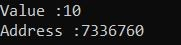That’s it for today. In the next article, I am going to discuss the Literals in C# with Examples. Here, in this article, I try to explain the Data Types in C# with Examples. I hope you understood the need and use of data types and I would like to have your feedback about this article.

### 7 thoughts on “Data Types in C#”

1.Great article. Thank you so much

2.Good article however, line Name = “c:\Pranaya\Dotnettutorials\Csharp”; will not compile and will correctly indicate an unrecognised escape sequence, like \P does not exist.
I assume you rather meant Name = “c:\\Pranaya\\Dotnettutorials\\Csharp”;

Thanks,
—– Jean Buchnik

1.Hi Jean Buchnik,
Did you use @ verbatim literal in your code like below?
Name = @”c:\Pranaya\Dotnettutorials\Csharp”;

It should work.

3.saurabh barasiya

superb

4.What an explanation. This is the best site to learn C#.NET. Thanks to the author for explaining the concept in such a manner.

5.Very much helpful as well as huge content full document, thanks.

6.EDIT: Regarding the float, double and decimal numbers discussion:

Accuracy is not the difference between these numbers. Depending upon what was being described, these types might be equally accurate. The difference is precision. For example, double would be able to accurately represent the distance of a far flung galaxy, while decimal could not. Decimal provides for many more significant digits, and can therefore deliver more precision, this does not mean it is more accurate. Overall, it’s a great site – thank you!